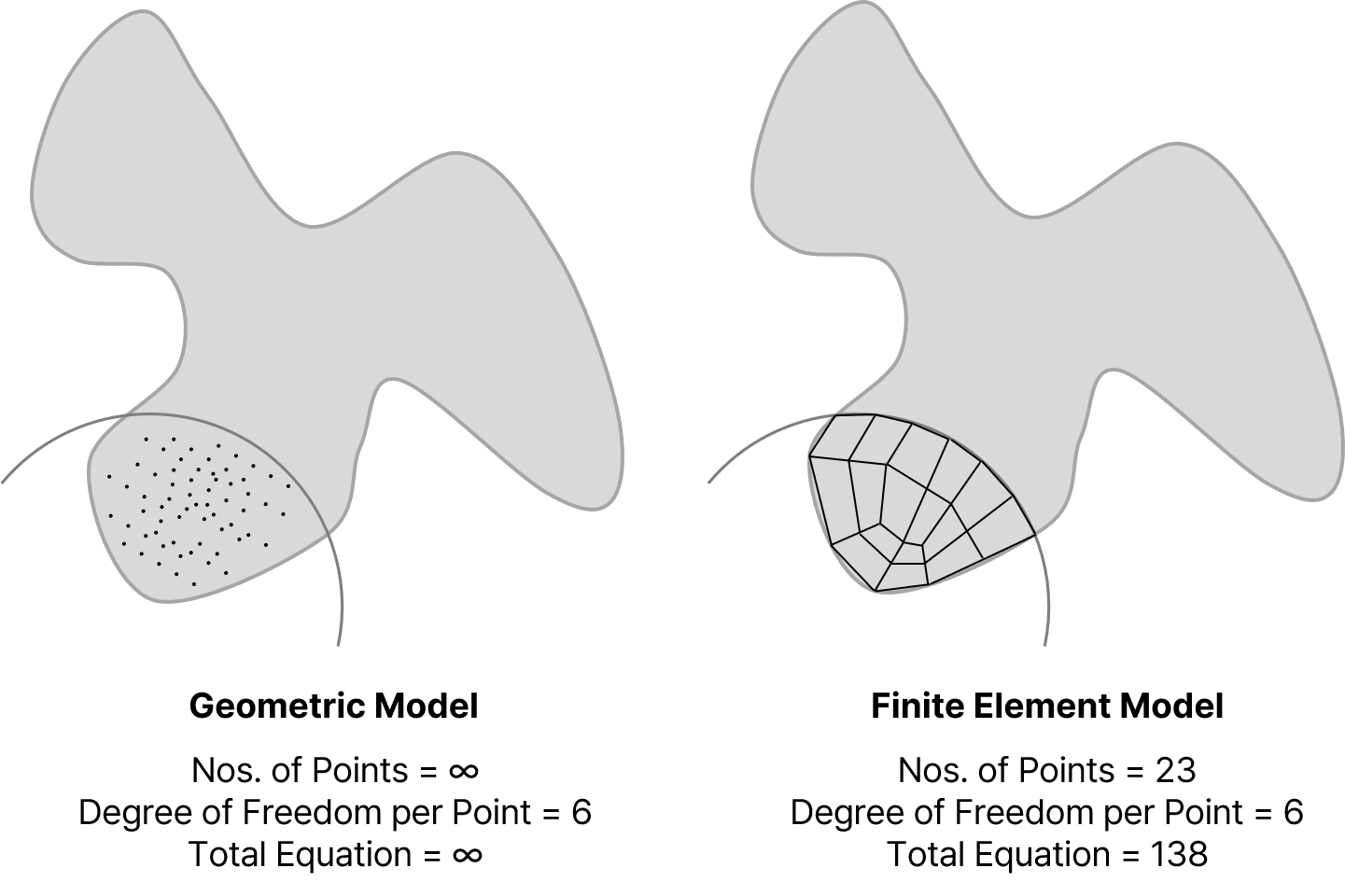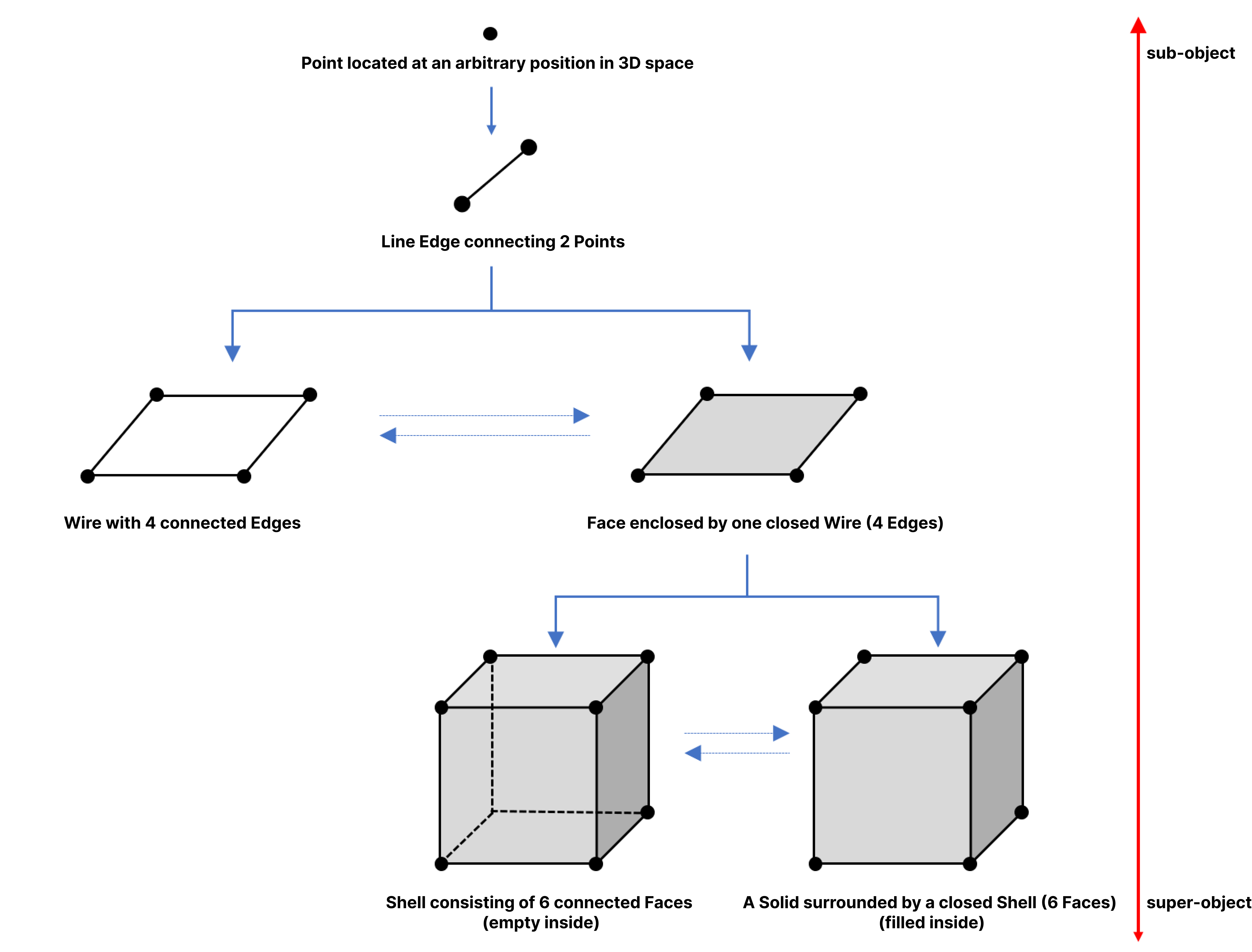BLOG NEWS & TRENDS

*Click the content to move to the section

## 1. Overview

There are various methods used in the calculation process for constructing structures, among which many structures are being performed through finite element analysis.

Using a simple 1D beam element in midas Civil, the overall structural system of a bridge is designed, and for more precise results, detailed design is carried out using midas Civil or FEA NX with 2D plate elements. For structures with complex shapes, or to verify more precise stress flow, analysis using a 3D solid model through midas Civil or FEA NX is conducted.

Even creating 1D and 2D elements takes a lot of time... Was it difficult to create a 3D solid model, which is also difficult to identify the shape through 2D drawings?

In this content, we will introduce the concept of model reusing, which is probably the most efficient method in the field of structural analysis among smart construction concepts.

## 2. Concept and Procedure of Finite Element Analysis (FEA)

Finite Element Analysis (FEA) is one of the computer simulation techniques used to perform complex numerical analysis. The common feature of various numerical analysis techniques is to approximate the mathematical representation (mostly differential equations) required for calculation by converting it into matrix form to obtain an approximate solution.

Although it may seem like a complex concept, it can be understood as a numerical analysis technique that simulates complex physical characteristics such as deformations caused by internal or external conditions in a structure within a physical system.

To perform these calculations, the geometric model consisting of infinite points and elements requires an infinite amount of operations to calculate the desired values. Therefore, we need to divide the geometric model into a finite number of elements, as shown in the figure below, to transform it into a finite element model so that the computer can determine the points required for the calculation.<Figure 1: Difference between Geometric Model and Finite Element Model>

As shown in the figure above, the more points (elements) there are, the more equations that need to be solved, which can lead to longer analysis times. Therefore, when creating a finite element model, modeling should be performed to minimize the number of elements.

The general procedure for conducting a finite element analysis is as follows:Figure 2. The procedure for finite element analysis

To perform Finite Element Analysis, it is necessary to create a geometry model and a finite element model in the pre-processing stage. While some programs, like Midas Civil, allow for direct creation of finite element models without a geometry model (Method 1), in other cases, a finite element model can be created from a geometry model by generating a mesh (Method 2).<Figure 3. Finite Element Model Generation Methods>

The geometric model used to create a finite element model contains only the shape information, without any structural analysis-related information. This type of model can incorporate curved shapes, providing greater freedom in modeling than a finite element model which must be created by combining elements made up of points and straight lines.

Therefore, while the geometric model can be modeled within a structural analysis program, it can also be created through various programs capable of performing 3D modeling, such as midas CIM. The models used in structural analysis typically have a hierarchical structure as shown below, which is applied equally to both the geometric model and the finite element model.<Figure 4. Hierarchy of Model Shapes>

Creating a geometric model using POINTs, LINEs, FACEs, and SOLIDs can be a surprisingly complex task. Rather than creating a geometric model from scratch for finite element analysis, it would be helpful to reuse an existing 3D model.

3. Model Reusing utilizing midas CIM and FEA NX

 💡 What is model reusing?   Model reusing in structural analysis refers to the technique of creating a new model by modifying an existing 3D solid model. This technique is used to save time and costs in the design and analysis of structures. Rather than creating a new model from scratch, certain portions of the existing model are modified to create a new design.   This process involves selecting the portion of the existing model that needs to be modified and considering the impact of these changes on the entire model. As a result, this technique requires a significant level of expertise and knowledge in the field of structural analysis.   Model reusing is a valuable technique for saving time and reducing errors in structural analysis. By reusing existing models, designers can make modifications more efficiently and accurately while achieving more precise results.

midas CIM provides various file formats for creating geometric models to assist engineers in their work. Among them, if the Parasolid format (.X_T) is used, it can be directly linked to FEA NX, making it more convenient to create finite element models using geometric models.

The following video shows the process of creating a finite element model for detailed analysis using the geometric model of the tendon anchorage part of the FCM bridge, which is part of the midas CIM Smart Template feature.

Video 1. Model Reusing (Utilizing MIDAS CIM and FEA NX)

As you can see in the video, it is possible to create a finite element model by applying material properties (using FEA NX's database) and element mesh generation characteristics to the 3D model that has already been created. Now, instead of creating multiple models for each analysis stage, you can reuse a well-made model to generate various models required for the overall structural analysis and detailed analysis.

Of course, FEA NX not only works with MIDAS CIM but also provides import and export functions for various formats such as Parasolid, ACIS, Step, Iges, Pro-E, and CATIA. Therefore, it is possible to work with most 3D modeling programs that can create 3D models.

The export and import functions of FEA NX allow engineers to use the 3D model program that they are most familiar with, making it easy to create finite element models for complex and special-shaped models.

Additionally, using MIDAS CIM, it is possible to create one-dimensional beam elements and two-dimensional plate elements required for structural analysis.

## 4. Conclusion

When performing finite element analysis, the most time-consuming task is likely to be creating the geometric model. It took a lot of time to understand the shape of the structure through the drawings, but now engineers can create finite element models that meet various analysis conditions by reusing existing 3D models. From now on, instead of creating a finite element model directly, use the 3D model created in the initial design stage.

Midas IT will continue to provide various ways to reduce your time and workload through content that matches the latest design trends.